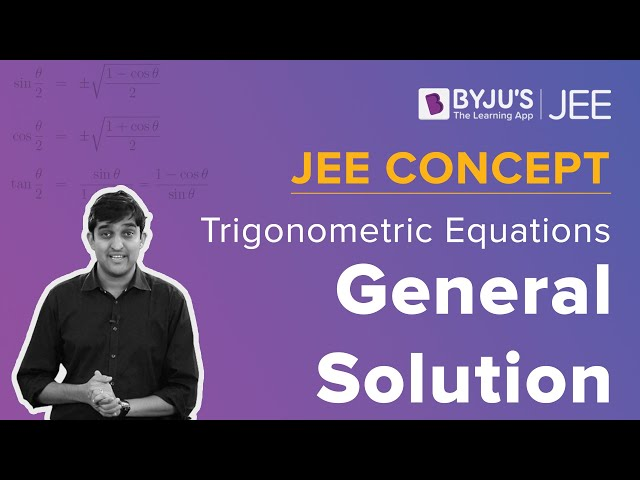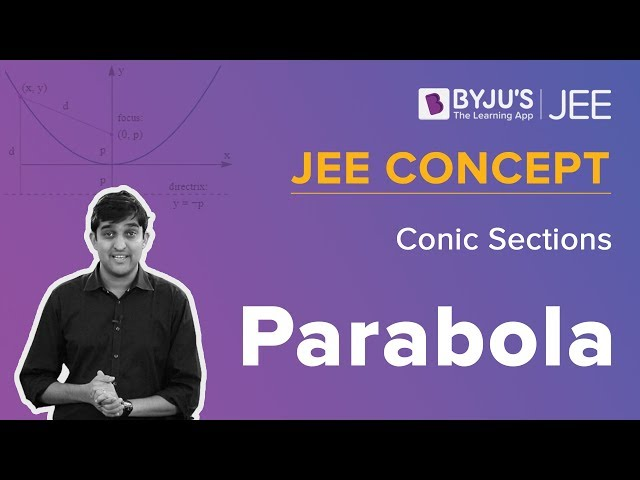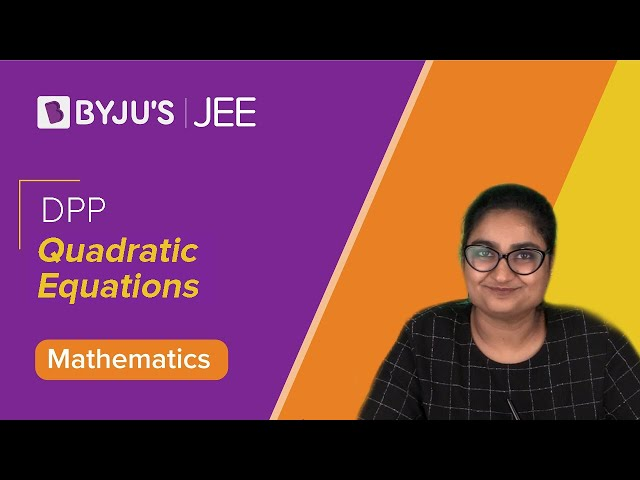Win up to 100% scholarship on Aakash BYJU'S JEE/NEET courses with ABNAT Win up to 100% scholarship on Aakash BYJU'S JEE/NEET courses with ABNAT

# JEE Advanced 2019 Maths Paper 2

Students preparing for JEE Advanced can refer 2019 solved Maths question paper 2 provided at BYJU’S. All the questions are solved by subject experts with detailed explanation. JEE Advanced question paper 2019 Maths Paper 2 solutions can also be downloaded to practise offline. Previous year question papers are always helpful for the students to evaluate their preparation level.
Paper 2 – Maths
Question 1. Let f: R -> R be given by f(x) = (x – 1) (x – 2) (x – 5). Define F(x) =
$$\begin{array}{l}\int_0^x f(t) dt, x > 0\end{array}$$
. Then which of the following options is/are correct?

a) F has a local minimum at x = 1
b) F has a local maximum at x = 2
c) F(x) ≠ 0 for all x ϵ (0, 5)
d) F has two local maxima and one local minimum in (0, ∞)

F(x) = (x – 1) (x – 2) (x – 5)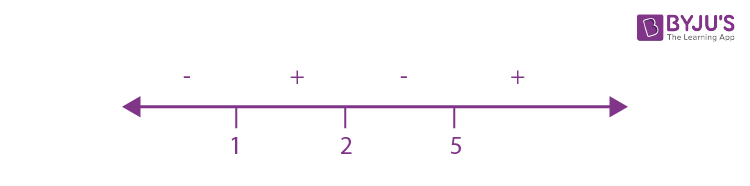At x = 1 and x = 5, F'(x) changes from – to +

Therefore, F(x) has two local minima points at x = 1 and x = 5.

F(x) has one local maxima point at x = 2.

Question 2.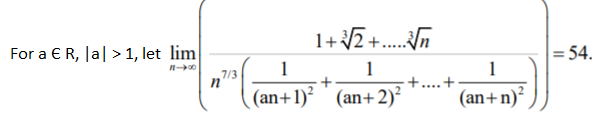Then the possible value(s) of a is/are:

a) 8
b) –9
c) –6
d) 7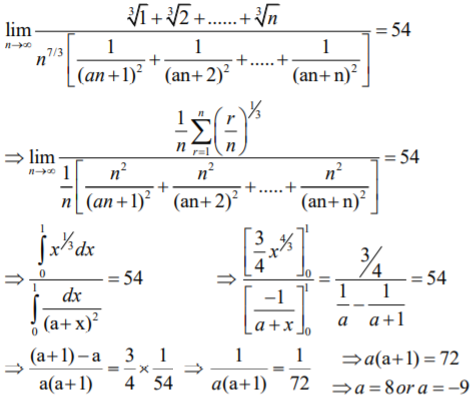Question 3: Three lines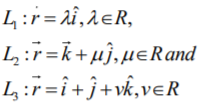are given. For which point(s) Q and L2 can we find a point P on L1 and a point R on L3 so that P, Q and R are collinear?

a)

$$\begin{array}{l}\hat k + \hat j\end{array}$$

b)
$$\begin{array}{l}\hat k\end{array}$$

c)
$$\begin{array}{l}\hat k + (1/2) \hat j\end{array}$$

d)
$$\begin{array}{l}\hat k – (1/2) \hat j\end{array}$$

P(λ, 0, 0), Q(0, μ, 1), R(1, 1, r)

Given:

$$\begin{array}{l}\vec {PQ} = k . \vec {PR}\end{array}$$

λ/[ λ-1] = – μ/-1 = -1/-r

μ cannot take the values 0 and 1.

Question 4: Let F: R -> R be a function. We say that f has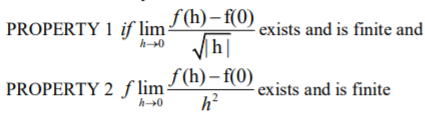Then which of the following options is/are correct?

a) f(x) = x|x| has PROPERTY 2
b) F(x) = x2/3 has PROPERTY 1
c) f(x) = sin x has PROPERTY 2
d) f(x) = |x| has PROPERTY 1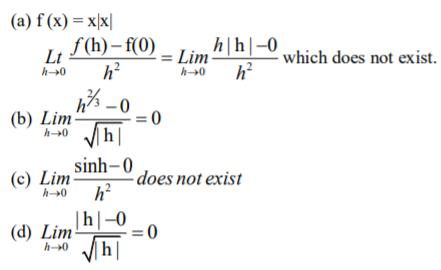Question 5: For non-negative integers n, let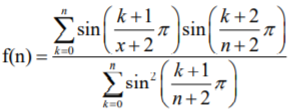Assuming cos-1x takes value in [0, π], which of the following options is/are correct?

a) If α = tan (cos-1f(6)), then α2 + 2α – 1 = 0
b) lim_(n-> ∞) f(n) = 1/2
c) f(4) = √3/2
d) sin (7 cos-1f(5)) = 0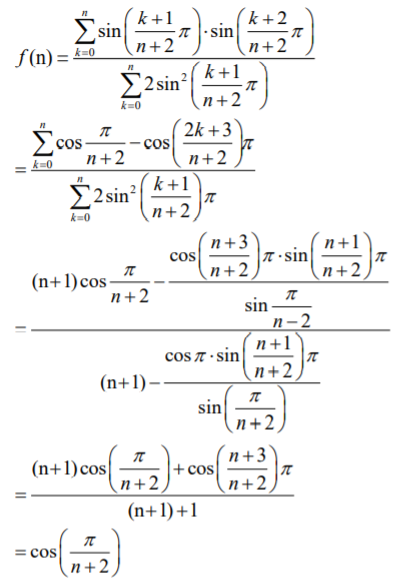(a) α = tan(cos-1 f(6)) = tan(cos-1(cos π/8) = tan (π/8)

α2 + 2 α – 1 = tan2 (π/8) + 2 tan (π/8) – 1

tan 2(π/8) = [2 tan (π/8)]/[1- tan2(π/8)]

This implies, 1 = 2α/[1- α2]

α2 + 2 α – 1 = 0

Option (a) is correct.

(b)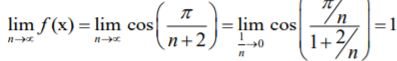Option (b) is not correct.

(c) f(4) = cos(π/6) = √3/2

Option (c) is correct.

(d) sin(7 cos-1 f(5)) = sin(7 cos-1 (cos π/7)) = sin(7 x π/7) = 0

Option (d) is correct.

Question 6: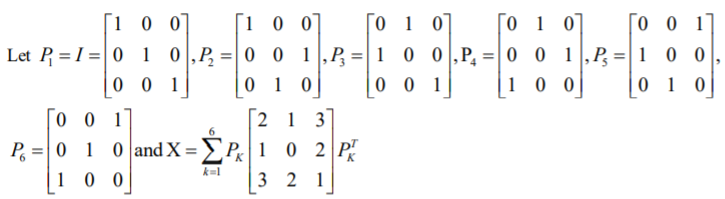Where PKT denotes the transpose of the matrix PK. Then which of the following options is/are correct?

a) X – 30I is an invertible matrix
b) The sum of diagonal entries of X is 18
c) If

$$\begin{array}{l}X \begin{bmatrix} 1\\ 1\\ 1 \end{bmatrix} = \alpha \begin{bmatrix} 1\\ 1\\ 1 \end{bmatrix}\end{array}$$
, then α = 30

d) X is a symmetric matrix

P1 = P1T = P1-1

P2 = P2T = P2-1

…….

P6 = P6T = P6-1

And Let

$$\begin{array}{l}A = \begin{bmatrix} 2 &1 &3 \\ 1 & 0& 2\\ 3& 2 &1 \end{bmatrix}\end{array}$$

Here AT = A -> A is symmetric matrix

Now, XT = (P1AP1T + ……+ P6AP6T)T

= P1AP1T + ……+ P6AP6T

= X

Therefore, X is symmetric matrix.

Again,

Let B =

$$\begin{array}{l}\begin{bmatrix} 1\\ 1\\ 1 \end{bmatrix}\end{array}$$

XB = P1AP1TB + P2AP2TB +……..+ P6AP6TB

= P1AB + P2AB+ ……+ P6AB

= (P1 + P2 + ……+ P6)

$$\begin{array}{l}\begin{bmatrix} 6\\ 3\\ 6 \end{bmatrix}\end{array}$$

=

$$\begin{array}{l}\begin{bmatrix} 30\\ 30\\ 30 \end{bmatrix}\end{array}$$
= 30 B

=> α = 30

Since

$$\begin{array}{l}X \begin{bmatrix} 1\\ 1\\ 1 \end{bmatrix} = 30 \begin{bmatrix} 1\\ 1\\ 1 \end{bmatrix}\end{array}$$

=>(X – 30I)B = 0 has a nontrivial solution B =

$$\begin{array}{l}\begin{bmatrix} 1\\ 1\\ 1 \end{bmatrix}\end{array}$$

=>X – 30I = 0

X = P1AP1T + …..+ P6AP6T

Trace(X) = tr(P1AP1T) + …..+ tr(P6AP6T)

= (2 + 0 + 1) + ……(2 + 0 + 1) = 3 + 3 + ….6 times = 18

Question 7: Let x ϵ R and let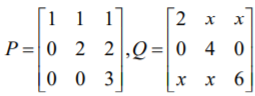And R = PQP-1. Then which of the following options is/are correct?

a) For x = 1, there exists a unit vector

$$\begin{array}{l}\alpha \hat i + \beta \hat j + \gamma \hat k \ for \ which \ R \begin{bmatrix} \alpha\\ \beta\\ \gamma \end{bmatrix} = \begin{bmatrix} 0\\ 0\\ 0 \end{bmatrix}\end{array}$$

b) There exists a real number x such that PQ = QP
c) det R =
$$\begin{array}{l}det\begin{bmatrix} 2 & x & x\\ 0& 4 & 0\\ x&x & 5 \end{bmatrix} + 8\end{array}$$
, for all x Є R.

d) For x = 0, if
$$\begin{array}{l}R\begin{bmatrix} 1\\ a\\ b \end{bmatrix} = 6\begin{bmatrix} 1\\ a\\ b \end{bmatrix}\end{array}$$
, then a + b = 5.

R = PQP-1

|R| = |P||Q||P-1|

=>det Q = 2(24) – x(0) + x(-4x) = 48 – 4x2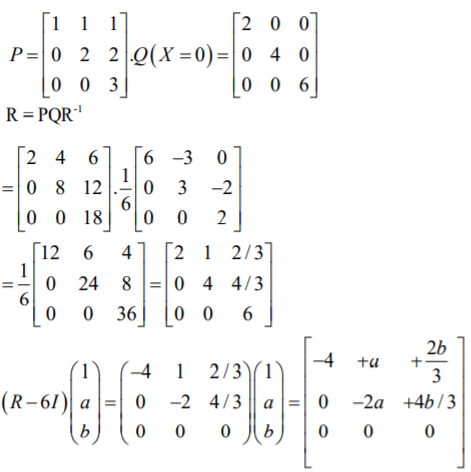-4 + a + 2b/3 = 0 and -2a + 4b/3 = 0 => a = 2 and b = 3

Therefore, a + b = 5

PQ = QP

=>x + 2 + x = 2 + 2x ->No value exists.

Question 8: Let f(x) = sinπx/x2 , x > 0

Let x1 < x2 < x3 <…..< xn < …. be all the points of local maximum of f.

and y1 < y2 < y3 <…..< yn < …. be all the points of local minimum of f.

Then which of the following options is/are correct?

a) |xn – yn| > 1 ;for every n
b) x1 < y1
c) xn Є (2n, 2n + 1/2) ;for every n
d) xn+1 – xn > 2 ;for every n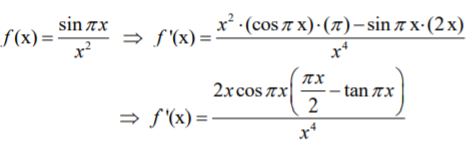*for students, Draw a graph and conclude.

Question 9: The value of
$$\begin{array}{l}sec^{-1}(1/4 \sum_{k=0}^{10} sec(7\pi/12 + k\pi/2))sec(7\pi/12 + (k+1)\pi/2))\end{array}$$
in the interval [-π/4, 3π/4] equals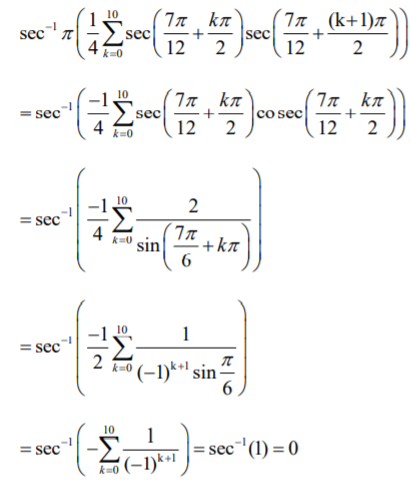Question 10: Let |X| denote the number of elements in set X. Let S = {1,2,3,4,5,6} be a sample space, where each element is equally likely to occur. If A and B are independent events associated with S, then the number of ordered pairs (A,B) such that 1 < |B| < |A|, equals.

The number of ordered pairs of (A, B) are

6C1(6C2 + 6C3 + 6C4 +…..+6C6 )+ 6C2 (6C3 + 6C4 + …+ 6C6) + 6C3 (6C4 + 6C5 +6C6) + 6C4 (6C5 + 6C6) + 6C5 . 6C6

= (6C1 . 6C2 + 6C1 . 6C3 +…..+ 6C1 . 6C6 )+ (6C2 . 6C3 + 6C2 . 6C4 +…..+ 6C2 . 6C6) + (6C3 . 6C4 + 6C3 . 6C5 + 6C3 . 6C6) + 6C4 . 6C5 + 6C4. 6C6 + 6C5 . 6C6

= (12C56C1) + (12C46C2) + (12C36C3) + (12C26C4) + (12C16C5)

= (12C1 + 12C2 + 12C3 + 12C4 + 12C5 ) – ( 6C1 + 6C2 + 6C3 + 6C4 + 6C5)

= 1585 – 62 = 1523

Question 11: Five person A, B, C, D and E are seated in a circular arrangement. If each of them is given a hat of one of the three colours red, blue and green, then the number of ways of distributing the hats such that the persons seated in adjacent seats get different coloured hats is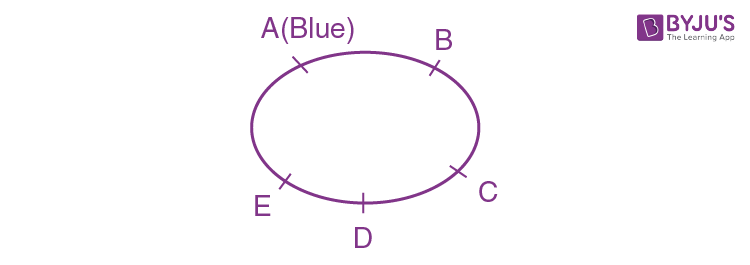Maximum number of hats used of same colour are 2.

They cannot be 3 otherwise atleast 2 hats of same colour are consecutive.

Now the hats used are consider as B B G G R

Which can be selected in 3 ways.

It can be R G G B B or R R G B B or G G R R B

The number of ways of distributing blue hat (single one) in 5 persons equal to 5.

Now either position B and D are filled by green hats and C and E are filled by Red hats or B & D are filled by Red hats and C & E are filled by Green hats.

So, only 2 ways are possible.

Hence, number of ways = 3×5×2 = 30 ways.

Question 12: Suppose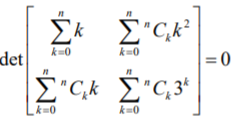holds for some positive integer n. Then

$$\begin{array}{l}\sum_{k=0}^n \frac{^nC_k}{k+1}\end{array}$$
equals.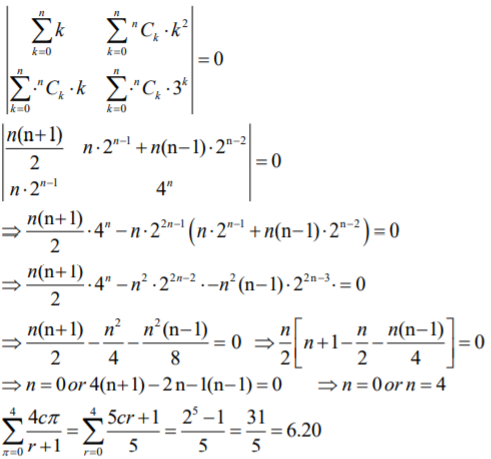Question 13: The value of the integral
$$\begin{array}{l}\int_0^{\frac{\pi}{2}} \frac{3 \sqrt{cos \theta}}{(\sqrt{cos \theta} + \sqrt{sin \theta})^5} \ d \theta\end{array}$$
equals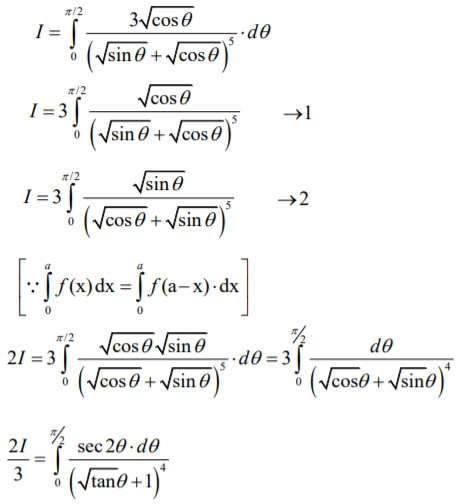Let tan θ = t2 =>sec 2θ dθ = dt

2I/3 =

$$\begin{array}{l}\int_0^{\infty} \frac{2tdt}{(t+1)^4}\end{array}$$

Hint: Split 2t/((t+1)4 = 1/(t+1)3 – 1/(t+1)4

Solving above integration, we get

I = 1/2

Question 14: Let
$$\begin{array}{l}\vec a = 2 \hat i + \hat j – \hat k \ and \ \vec b = \hat i + 2 \hat j + \hat k\end{array}$$
be two vectors. Consider a vector
$$\begin{array}{l}\vec c = \alpha \vec a + \beta \vec b , \alpha, \beta \in R\end{array}$$
. If the projection of vector c on the vector (a+b) is 3√2, then the minimum value of
$$\begin{array}{l}(\vec c – (\vec a \times \vec b). \vec c)\end{array}$$
equals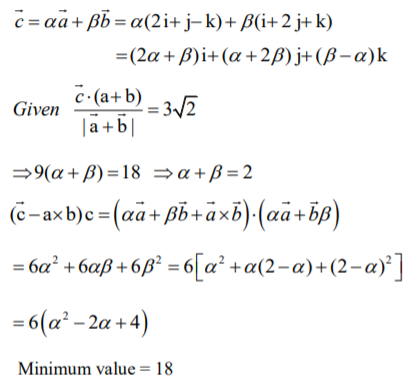Question 15: Answer the following by appropriately matching the lists based on the information given in the paragraph

Let f(x) = sin(π cosx) and g(x) = cos(2π sinx) be two functions defined for x > 0. Define the following sets whose element are written in the increasing order:

X = {x: f(x) = 0}, Y = {x: f'(x) = 0}

Z = {x: g(x) = 0}, W = {x: g'(x) = 0}

List –I contains the sets X,Y,Z and W. List – II contains some information regarding these sets.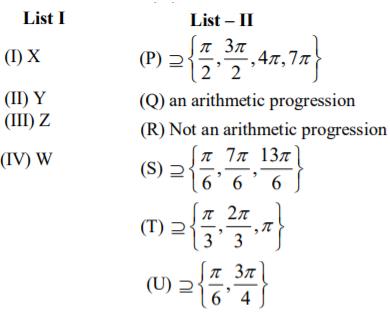Which of the following is the only correct combination?

a) (II), (R), (S)
b) (I), (P), (R)
c) (II), (Q), (T)
d) (I), (Q), (U)

Note: For answer, check next solution.

Question 16: Answer the following by appropriately matching the lists based on the information given in the paragraph.

Let f(x) = sin(π cosx) and g(x) = cos(2π sinx) be two functions defined for x > 0. Define the following sets whose element are written in the increasing order:

X = {x: f(x) = 0}, Y = {x: f'(x) = 0}

Z = {x: g(x) = 0}, W = {x: g'(x) = 0}

List –I contains the sets X,Y,Z and W. List – II contains some information regarding these sets.Which of the following is the only correct combination?

a) (IV), (Q), (T)
b) (IV), (P), (R), (S)
c) (III), (R), (U)
d) (III), (P), (Q), (U)

f(x) = 0

=> sin(π cos x) = 0

This implies, π cos x = nπ

Cos x = n

=>Cos x = -1, 0, 1

x = {nπ , (2nπ) π /2}

=> x = {nπ/2, n Є I}

f’(x) = 0

=> cos(π cos x)(- π sin x) = 0

=> π cos x = (2n + 1) π/2 or x = nπ

=> cos x = n + (1/2) or x = nπ

=> cos x = ±(1/2) or x = nπ

Therefore, y = {2nπ ± π/3, 2nπ ± 2π/3, n π }

g(x) = 0 => cos (2 π sin x) = 0

=> 2 π sin x = (2n + 1) π/2

=> sin x = (2n+1)/4 = ±(1/4), ±(3/4)

z = {nπ ± sin-1(1/4), nπ ± sin-1(3/4), n Є I}

g’(x) = 0 => -sin (2 π sin x) 2 π cos x = 0

=> 2 π sin x = n π or x = (2n + 1) π/2

=> sin x = n/2 = 0, ±(1/2), ±1 or x = (2n + 1) π/2

And, w = {nπ, (2n + 1) π/2, nπ ± π/6, n Є I}

Question 17: Answer the following by appropriately matching the lists based on the information given in the paragraph.

Let the circles C1 : x2 + y2 = 9 and C2 : (x – 3) 2 + (y – 4) 2 = 16, intersect at the points X and Y. Suppose that another circle C3 : (x – h) 2 + (y – k) 2 = r2 satisfies the following conditions:

(i) centre of C3 is collinear with the centres of C1 and C2

(ii) C1 and C2 both lie inside C3, and

(iii) C3 touches C1 at M and C2 at N

Let the line through X and Y intersect C3 at Z and W, and let a common tangent of C1 and C3 be a tangent to the parabola x2 = 8αy.

There are some expressions given in the List – I whose values are given in List – II below: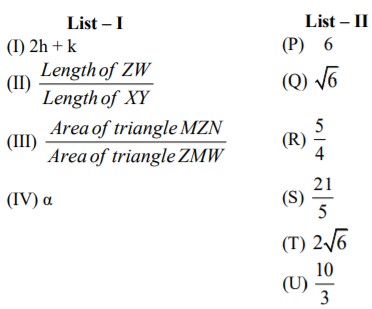Which of the following is the only INCORRECT combination?

a) (IV), (S)
b) (IV), (U)
c) (III), (R)
d) (I), (P)

(i) C1 , C2 and C3 are collinear.

$$\begin{array}{l}\begin{vmatrix} 0 &0 &1 \\ 3 & 4 &1 \\ h & k &1 \end{vmatrix}=0\end{array}$$

3k = 4h….(a)

Diameter of C3 = 12, so radius = 6 …(b)

C3 touches C1

C1(0, 0) and C3(h, k)

|C1C3| = |r – 3|

So, h2 + k2 = 9…(c)

From (a) and (c)

h = ±9/5 and k = ±12/5

[Neglect -ve values]

=> 2h + k = 6

(ii) Equation of line ZW

C1 = C2

=> 3x + 4y = 9

=> Distance of ZW from (0, 0)

$$\begin{array}{l}|\frac{-9}{\sqrt{3^2 + 4^2}}| = \frac{9}{5}\end{array}$$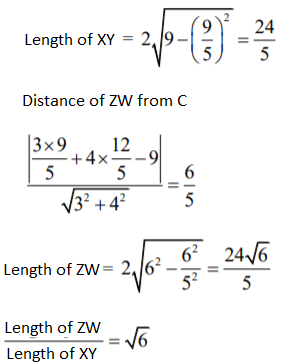(ii) Area of ΔmZN = (1/2). Nm. (1/2 . ZW) = 72√6/5

Area of ΔZmW = (1/2). ZW . (om + op) = (1/2) . 24√6/5 . (3 + 9/5) = 288√6/25

Therefore, [Area of ΔmZN]/ [Area of ΔZmW] = 5/4

(iv) Slope of tangent to C1 at m = -3/4

Equation of Tangent, y = mx – 2√(1+m2)

y = -3x/4 – 3√[1+9/16] = -3x/4 – 15/4

x = -4y/3 – 5 …(i)

Tangent to x2 = 4 (2α) y is x = m’y = 2α/m’ …(ii)

On comparing (i) and (ii), we get

m’ = -4/3 and 2α/m’ = -5 => α = 10/3

Question 18: Answer the following by appropriately matching the lists based on the information given in the paragraph.

Let the circles C1 : x2 + y2 = 9 and C2 : (x – 3) 2 + (y – 4) 2 = 16, intersect at the points X and Y. Suppose that another circle C3 : (x – h) 2 + (y – k) 2 = r2 satisfies the following conditions:

(i) centre of C3 is collinear with the centres of C1 and C2

(ii) C1 and C2 both lie inside C3, and

(iii) C3 touches C1 at M and C2 at N

Let the line through X and Y intersect C3 at Z and W, and let a common tangent of C1 and C3 be a tangent to the parabola x2 = 8αy.

There are some expressions given in the List – I whose values are given in List – II below:Which of the following is the only INCORRECT combination?

a) (II), (T)
b) (I), (S)
c) (I), (U)
d) (II), (Q)

*Note: Refer below solution.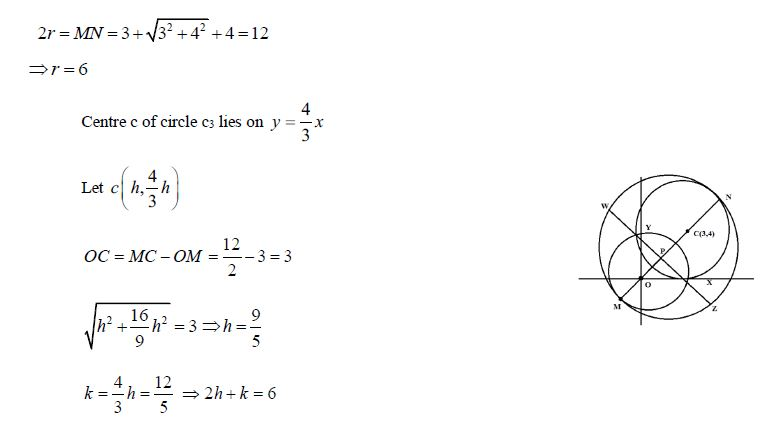## Video Lessons – Paper 2 Maths